Next: Minute No. 2, the Up: Quantum Field Theory in Previous: Quantum Field Theory in

Minute No. 1, the Classical Mechanics

Classical systems Gol53 are described by defining two elements: 1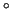- the set of classical coordinates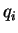, which are supposed to give a complete information about the state of the system, and 2- the Lagrangian. The state depends on a parameter called the classical absolute time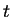, and hence, coordinates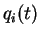are functions of time. The Lagrangian,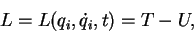(1)

is a function of coordinates, velocities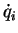, and time. According to the mechanistic point of view of the classical mechanics, every system in our Universe, including the whole Universe, is fully described by finding its coordinates and Lagrangian. For most systems the Lagrangian is equal to a difference of the kinetic energy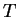, depending only on velocities, and the potential energy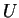, depending only on coordinates [see the second member of Eq. (1)].

Once the system is defined as above, its properties can be derived from simple principles. The time evolution of the system can be found from the principle of extremal action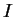,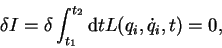(2)

which gives the Euler-Lagrange equations,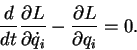(3)

This leads to a set coupled differential equations that can be, in principle, solved once the initial conditions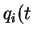=0) and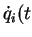=0) are known. One thus obtains the complete past and future history of the system. The rest is just a technicality ;) of how to solve differential equations. For typical systems, the kinetic energy is a quadratic function of velocities, for which the Euler-Lagrange equations are linear - and can be solved fairly easily.

Although we do not really need it in classical mechanics, we shell also introduce the formulation in terms of the Hamiltonian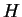. This gives us a bridge towards the quantum mechanics. Namely, we define the classical momentum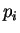by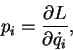(4)

and we transform the Lagrangian into the Hamiltonian,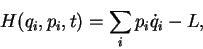(5)

as well as the Euler-Lagrange equations into the Hamilton equations,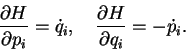(6)Next: Minute No. 2, the Up: Quantum Field Theory in Previous: Quantum Field Theory in
Jacek Dobaczewski 2003-01-27